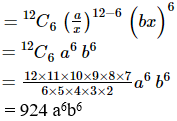Find the middle term in the expansion of $$(\dfrac{a}{x} + bx)^{12}$$

Asked by Sakshi | 1 year ago |  63

##### Solution :-

We have,

where, n = 12 (even number)

So the middle term is ($$\dfrac{n}{2}$$ + 1)

= ($$\dfrac{12}{2}$$ + 1)

= (6 + 1) = 7. ie., 7th term

Now,

T7 = T6+1= 924 a6b6

Hence, the middle term is 924 a6b6.

Answered by Sakshi | 1 year ago

### Related Questions

#### Find the term independent of x in the expansion of (3/2 x2 – 1/3x)9

Find the term independent of x in the expansion of $$(\dfrac{3}{2x^2} – \dfrac{1}{3x})^9$$

#### Find the middle term in the expansion of (x – 1/x)2n+1

Find the middle term in the expansion of $$(x-\dfrac{ 1}{x})^{2n+1}$$

#### Find the middle term in the expansion of (1 + 3x + 3x2 + x3)2n

Find the middle term in the expansion of (1 + 3x + 3x2 + x3)2n

Find the middle term in the expansion of $$(\dfrac{x}{a} – \dfrac{a}{x})^{10}$$